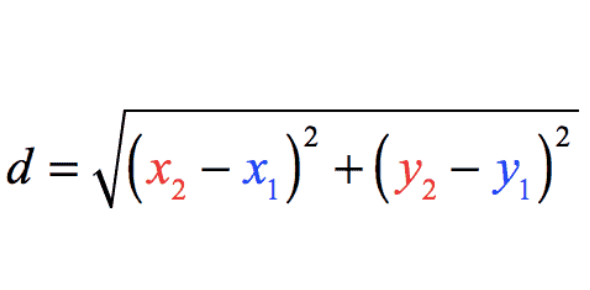# Distance Formula Quiz Questions And Answers

10 Questions | Attempts: 1516
ShareSettingsAre you looking out to practice the distance formula? You can take this distance formula quiz to check yourself on the basics of distance formula with these questions and answers. As the distance formula is among the basics of coordinate geometry, it is important to learn and practice. Go ahead with this practice test, and get a perfect score. Even if you miss out on something, we will help you with the correct answer. All the best! You can share the quiz with others too.

• 1.
The distance from (1, 0) to (x, 0) is equal to 1. While there is more than one possible x-coordinate for the second point, which of the following options satisfies the distance formula with the given coordinates?
• A.

-1

• B.

2

• C.

4

• D.

0

• 2.
Distance can be negative.
• A.

True

• B.

False

• 3.
The distance from (6,7) to (8,9) is equal to (leave your answer in a simplified radical form - i.e., no decimals)
• A.

units

• B.

units

• C.

units

• D.

units

• 4.
The distance from (-1,-2) to (3,4) is equal to (leave your answer in a simplified radical form - i.e., no decimals)
• A.

units

• B.

units

• C.

units

• D.

units

• 5.
Which of the following is an appropriate label for distance?
• A.

Milliliters

• B.

Kilometers

• C.

Square inches

• D.

Pounds

• 6.
Which of the following is NOT an appropriate label for distance?
• A.

Joules

• B.

Meters

• C.

Feet

• D.

Yards

• 7.
The distance from (2,-2) to (5,2) is equal to (leave your answer in a simplified radical form - i.e., no decimals)
• A.

units

• B.

units

• C.

units

• D.

units

• 8.
Which of the following equations represents the distance formula? (Hint: there is more than one correct answer)
• A.
• B.
• C.
• D.
• E.
• 9.
The distance from (-1,4) to (4, -1) is equal to (leave your answer in a simplified radical form - i.e., no decimals)
• A.

units

• B.

units

• C.

units

• D.

units

• 10.
Find the distance between the two points (4, 1) and (10, 9) (leave your answer in a simplified radical form - i.e., no decimals)
• A.

units

• B.

10 units

• C.

64 units

• D.

units

## Related TopicsBack to top
×

Wait!
Here's an interesting quiz for you.